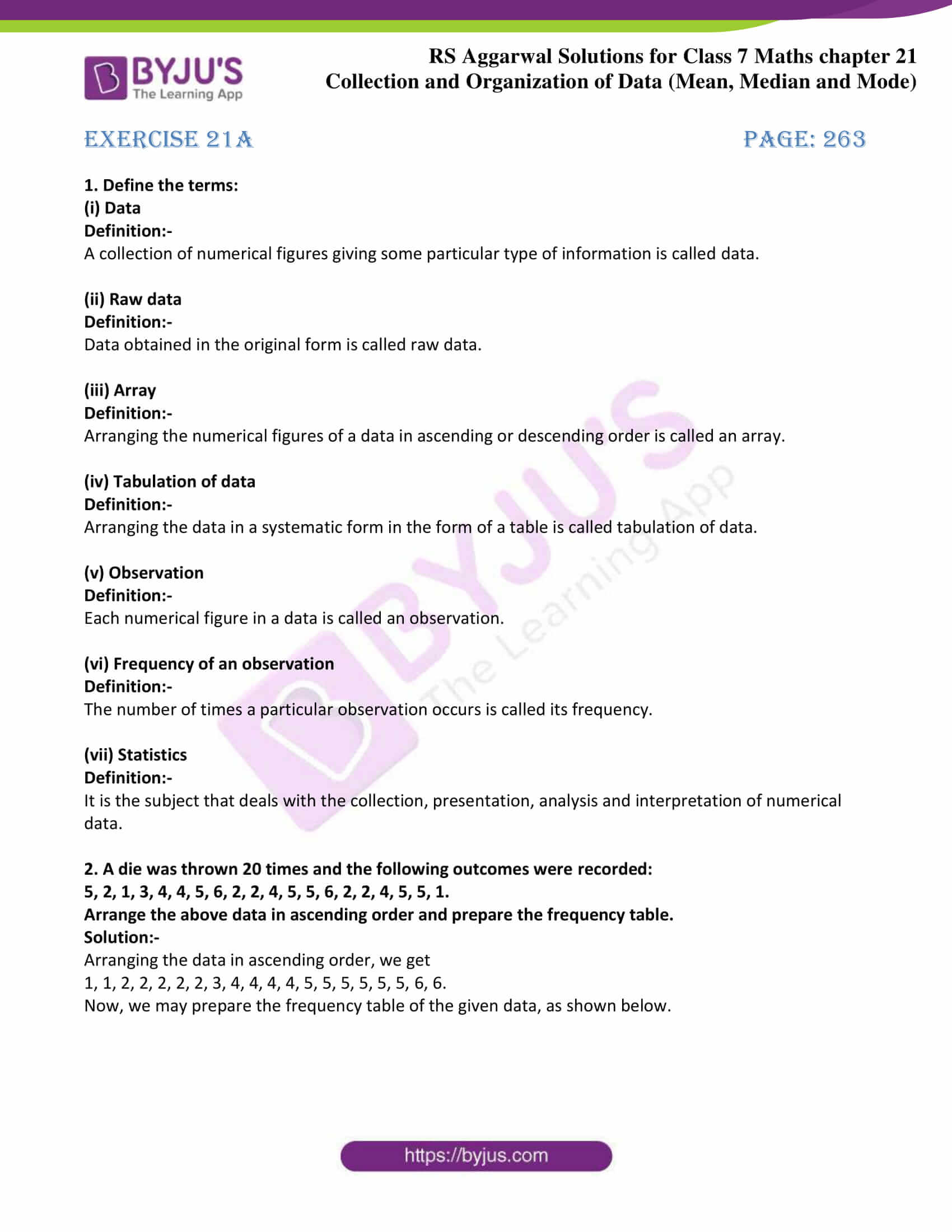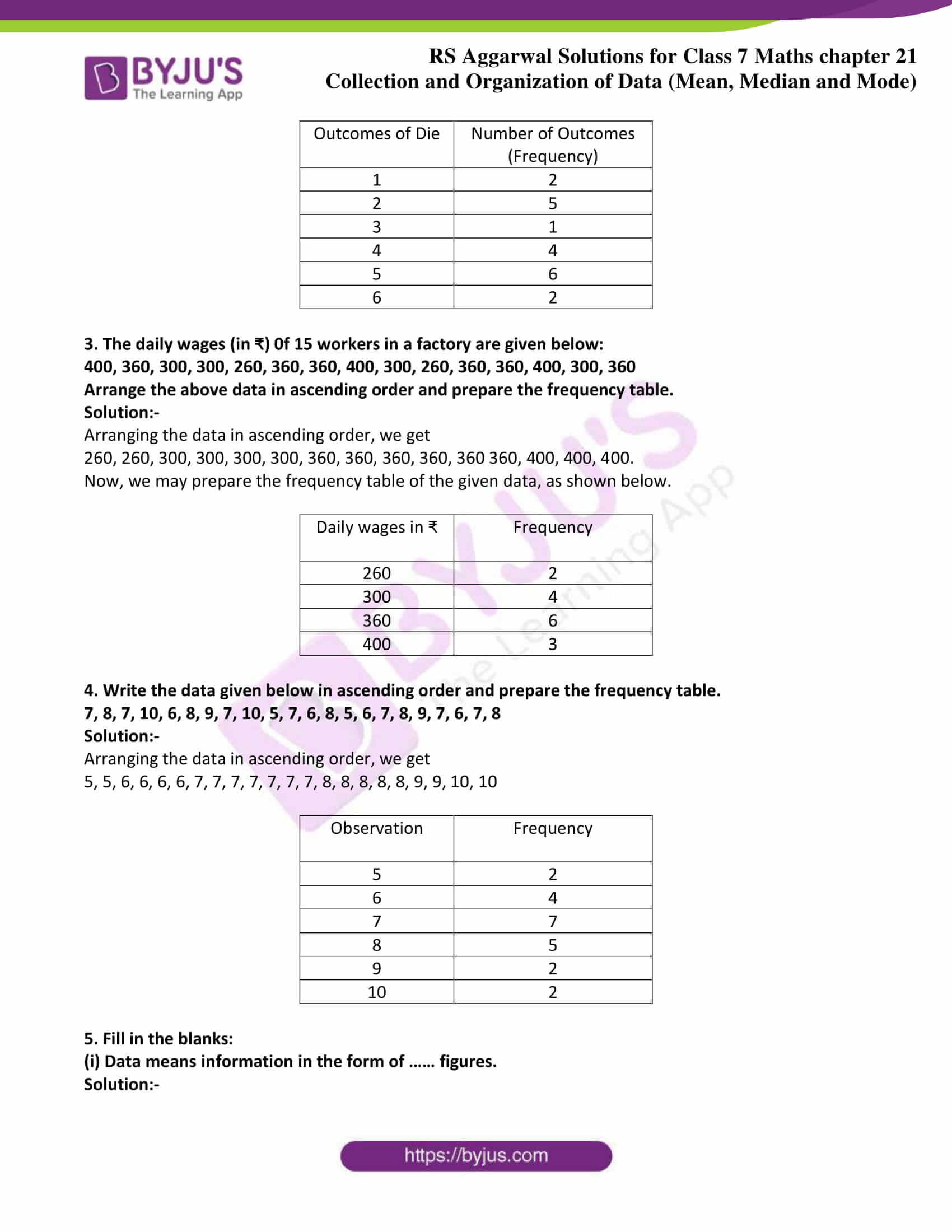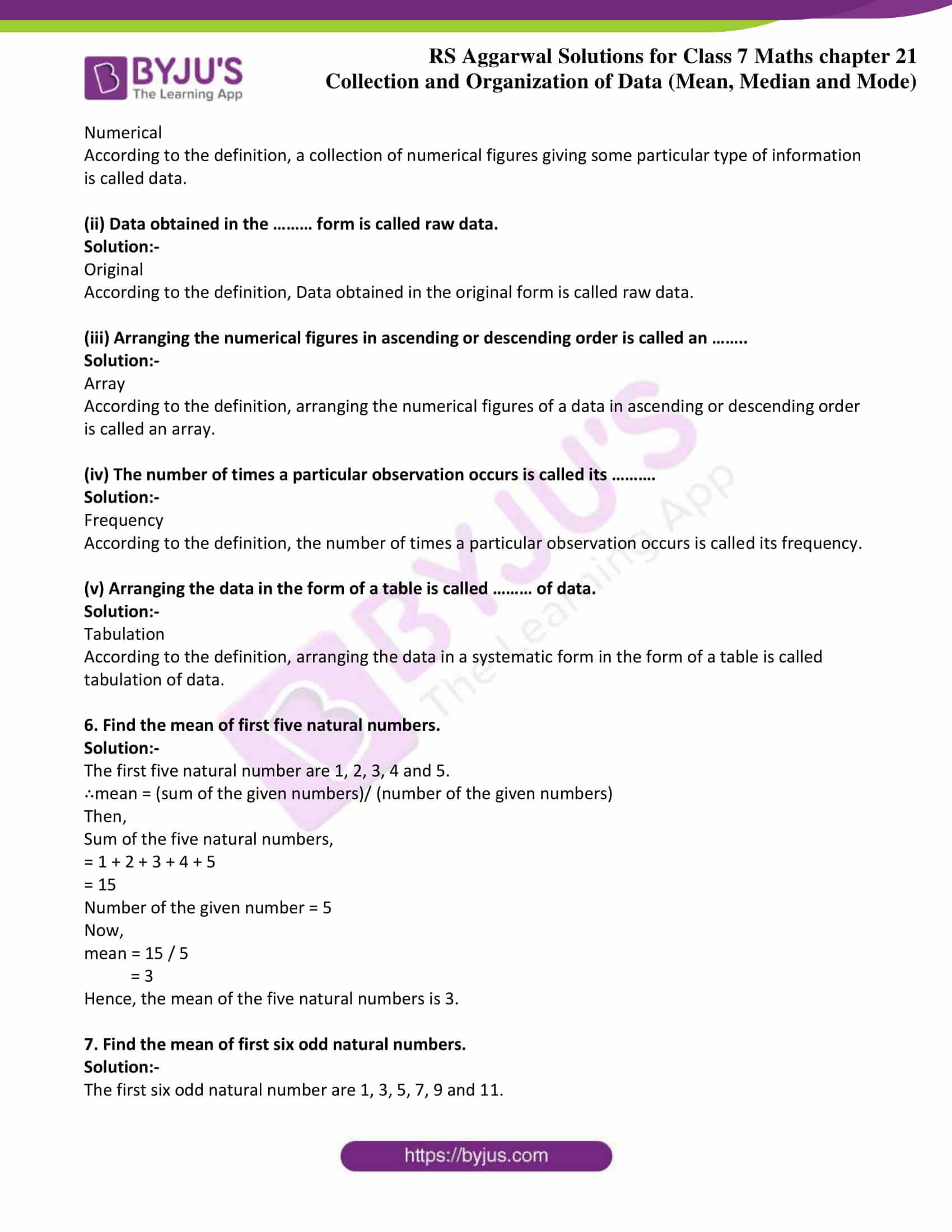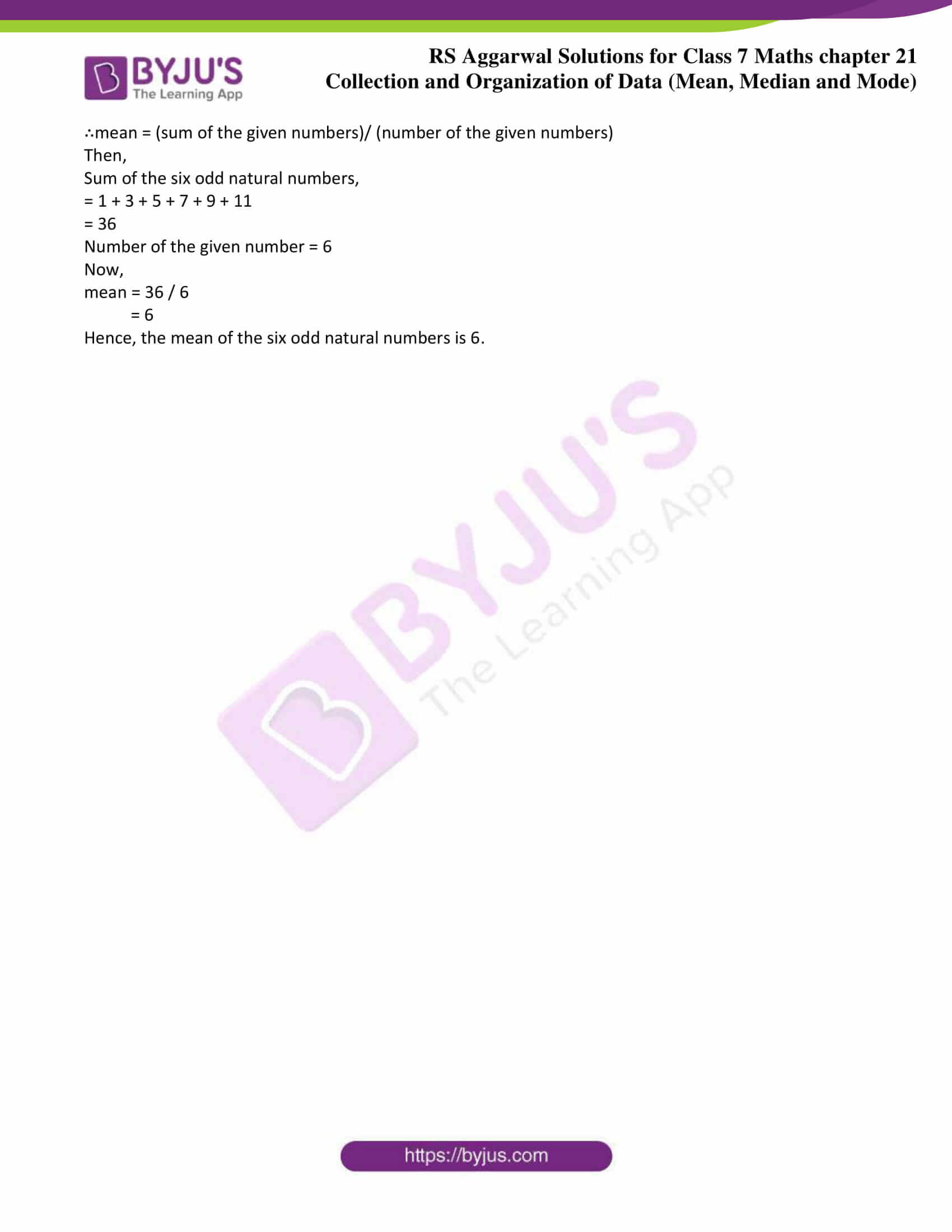# RS Aggarwal Solutions for Class 7 Maths Exercise 21A Chapter 21 Collection and Organisation of Data (Mean, Median and Mode)

The simple PDF of RS Aggarwal Solutions for the Exercise 21A of Class 7 Maths Chapter 21 Collection and Organisation of Data (Mean, Median and Mode) is the best study material. It provides solutions to all the questions present in the RS Aggarwal Solutions for Exercise 21A of Class 7 Maths Chapter 21. Topics covered in this exercise are mean of ungrouped data and mean of tabulated data. By the definition, Mean is defined as the sum of the given observations by a number of the given observations. Students can refer to these RS Aggarwal Class 7 Solutions Chapter 21 and practice various questions daily to perform best in the final exams.

## Download the PDF of RS Aggarwal Solutions For Class 7 Maths Chapter 21 Collection and Organisation of Data (Mean, Median and Mode) – Exercise 21A### Access answers to Maths RS Aggarwal Solutions for Class 7 Chapter 21 – Collection and Organisation of Data (Mean, Median and Mode) Exercise 21A

1. Define the terms:

(i) Data

Definition:-

A collection of numerical figures giving some particular type of information is called data.

(ii) Raw data

Definition:-

Data obtained in the original form is called raw data.

(iii) Array

Definition:-

Arranging the numerical figures of a data in ascending or descending order is called an array.

(iv) Tabulation of data

Definition:-

Arranging the data in a systematic form in the form of a table is called tabulation of data.

(v) Observation

Definition:-

Each numerical figure in a data is called an observation.

(vi) Frequency of an observation

Definition:-

The number of times a particular observation occurs is called its frequency.

(vii) Statistics

Definition:-

It is the subject that deals with the collection, presentation, analysis and interpretation of numerical data.

2. A die was thrown 20 times and the following outcomes were recorded:

5, 2, 1, 3, 4, 4, 5, 6, 2, 2, 4, 5, 5, 6, 2, 2, 4, 5, 5, 1.

Arrange the above data in ascending order and prepare the frequency table.

Solution:-

Arranging the data in ascending order, we get

1, 1, 2, 2, 2, 2, 2, 3, 4, 4, 4, 4, 5, 5, 5, 5, 5, 5, 6, 6.

Now, we may prepare the frequency table of the given data, as shown below.

 Outcomes of Die Number of Outcomes (Frequency) 1 2 2 5 3 1 4 4 5 6 6 2

3. The daily wages (in ₹) 0f 15 workers in a factory are given below:

400, 360, 300, 300, 260, 360, 360, 400, 300, 260, 360, 360, 400, 300, 360

Arrange the above data in ascending order and prepare the frequency table.

Solution:-

Arranging the data in ascending order, we get

260, 260, 300, 300, 300, 300, 360, 360, 360, 360, 360 360, 400, 400, 400.

Now, we may prepare the frequency table of the given data, as shown below.

 Daily wages in ₹ Frequency 260 2 300 4 360 6 400 3

4. Write the data given below in ascending order and prepare the frequency table.

7, 8, 7, 10, 6, 8, 9, 7, 10, 5, 7, 6, 8, 5, 6, 7, 8, 9, 7, 6, 7, 8

Solution:-

Arranging the data in ascending order, we get

5, 5, 6, 6, 6, 6, 7, 7, 7, 7, 7, 7, 7, 8, 8, 8, 8, 8, 9, 9, 10, 10

 Observation Frequency 5 2 6 4 7 7 8 5 9 2 10 2

5. Fill in the blanks:

(i) Data means information in the form of …… figures.

Solution:-

Numerical

According to the definition, a collection of numerical figures giving some particular type of information is called data.

(ii) Data obtained in the ……… form is called raw data.

Solution:-

Original

According to the definition, Data obtained in the original form is called raw data.

(iii) Arranging the numerical figures in ascending or descending order is called an ……..

Solution:-

Array

According to the definition, arranging the numerical figures of a data in ascending or descending order is called an array.

(iv) The number of times a particular observation occurs is called its ……….

Solution:-

Frequency

According to the definition, the number of times a particular observation occurs is called its frequency.

(v) Arranging the data in the form of a table is called ……… of data.

Solution:-

Tabulation

According to the definition, arranging the data in a systematic form in the form of a table is called tabulation of data.

6. Find the mean of first five natural numbers.

Solution:-

The first five natural number are 1, 2, 3, 4 and 5.

∴mean = (sum of the given numbers)/ (number of the given numbers)

Then,

Sum of the five natural numbers,

= 1 + 2 + 3 + 4 + 5

= 15

Number of the given number = 5

Now,

mean = 15 / 5

= 3

Hence, the mean of the five natural numbers is 3.

7. Find the mean of first six odd natural numbers.

Solution:-

The first six odd natural number are 1, 3, 5, 7, 9 and 11.

∴mean = (sum of the given numbers)/ (number of the given numbers)

Then,

Sum of the six odd natural numbers,

= 1 + 3 + 5 + 7 + 9 + 11

= 36

Number of the given number = 6

Now,

mean = 36 / 6

= 6

Hence, the mean of the six odd natural numbers is 6.

### Access other exercises of RS Aggarwal Solutions For Class 7 Chapter 21 – Collection and Organisation of Data (Mean, Median and Mode)

Exercise 21B Solutions

Exercise 21C Solutions